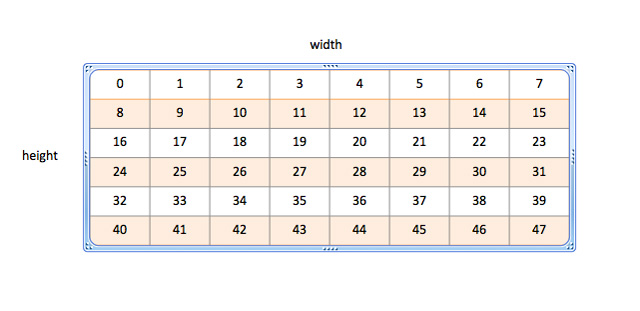# OpenCV and Processing 5

Now we proceed to first core part of the tutorials, representation of image in Processing and OpenCV. In Processing, the class is PImage. Digital video, Movie and live webcam feed, Capture are also PImage. When we import the external image file from the data folder through loadImage(), the format will usually be RGB. The internal representation is, however, ARGB.

The data is maintained in an integer linear array pixels[]. Each pixel is a 4 bytes color element.In the diagram, it is the representation of an image of size width (8) x height (6). The pixels are arranged from 0 to 47. Each cell is a color data element, and which is also an integer. The color element uses one byte to represent each elementary color, including alpha channel, in the order of ARGB.

The following sketch displays the color information for a random pixel of the PImage loaded from an external file in the data folder.

```PImage img;   void setup() { size(640, 480); background(0); img = loadImage("sample01.jpg"); noLoop(); }   void draw() { image(img, 0, 0); int x = floor(random(img.width)); int y = floor(random(img.height)); color c = img.pixels[y*img.width + x]; println("Alpha: " + alpha(c)); println("Red: " + red(c)); println("Green: " + green(c)); println("Blue: " + blue(c)); }```